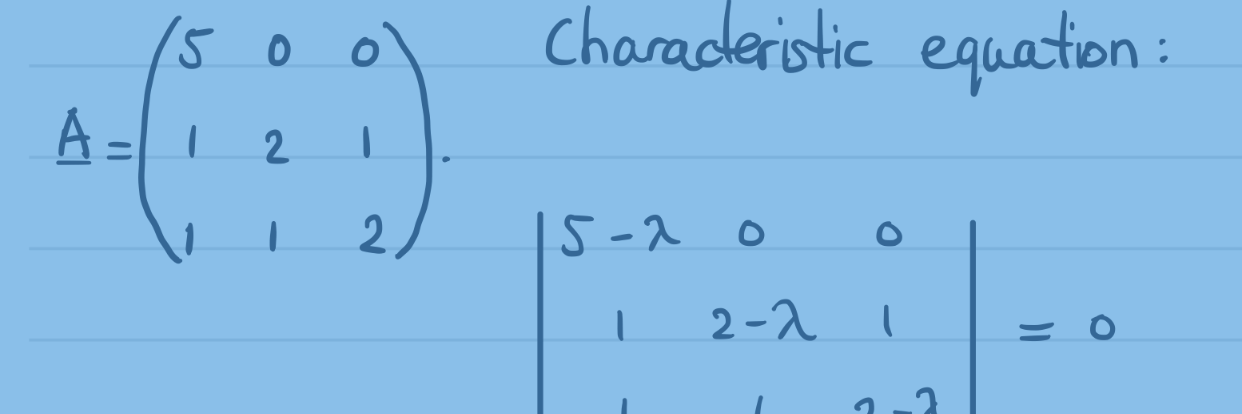# Finding the normal force in planar non-uniform circular motion using polar coordinates

We find an expression for the normal force on a mass which is in planar non-uniform circular motion using polar coordinates. Continue reading Finding the normal force in planar non-uniform circular motion using polar coordinates# Deriving the volume of the inside of a sphere using spherical coordinates

Using a volume integral and spherical coordinates, we derive the formula of the volume of the inside of a sphere, the volume of a ball. Continue reading Deriving the volume of the inside of a sphere using spherical coordinates# Simple problems on relativistic energy and momentum

We will focus on a few simple problems where we will manipulate Einstein’s equations for relativistic energy and momentum. Continue reading Simple problems on relativistic energy and momentum# Simple problems on relativistic energy and momentum

We will focus on a couple of simple problems where we will manipulate the equations for relativistic energy en momentum. Continue reading Simple problems on relativistic energy and momentum# Deriving the Lorentz transformations from a rotation of frames of reference about their origin with real time Wick-rotated to imaginary time

Well-known for their central role in Einstein’s Special Relativity, the Lorentz transformations are derived from the rotation of two frames of reference in standard configuration while time is taken to be an imaginary unit of spacetime. This is rarely seen in the wild. Not many undergraduate textbooks or online texts show the details of the working. Hence, this article. Continue reading Deriving the Lorentz transformations from a rotation of frames of reference about their origin with real time Wick-rotated to imaginary time# Real eigenvalues and eigenvectors of 3×3 matrices, example 3

We calculate the eigenvalues and eigenvectors of a 3×3 matrix in real number space. Continue reading Real eigenvalues and eigenvectors of 3×3 matrices, example 3# Real eigenvalues and eigenvectors of 3×3 matrices, example 2

We calculate the eigenvalues and eigenvectors of a 3×3 matrix in real number space. Continue reading Real eigenvalues and eigenvectors of 3×3 matrices, example 2# Real eigenvalues and eigenvectors of 3×3 matrices, example 1

We calculate the eigenvalues and eigenvectors of a 3×3 matrix in real number space. Continue reading Real eigenvalues and eigenvectors of 3×3 matrices, example 1